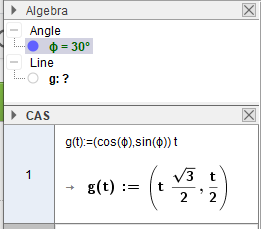# CAS Line undefined at angle of 30°

hawe shared this problem 2 years ago
New

Line undefined at angle of 30°1

Try g(t) = sin(ϕ) x / cos(ϕ)1

g = sin(ϕ) x / cos(ϕ) and not g(t)1

Ich arbeite im CAS und g(t) ist eine Gerade in Parameterform die für alle winkel definiert nur nicht für 30°1

Peut-être cela alors

Files: g(t).jpg1

g(t):=(t,t) is a function of R to R^2 for CAS

this has no sense in GG algebra. (t,t) is converted to X=(0,0)+t (1,1) that is a line in GG algebra

t (cos(phy),sin(phy)) is also converter if you type it in algebra view